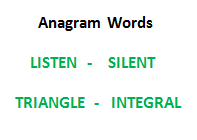GeeksforGeeks App
Open AppBrowser
Continue

# Check whether two Strings are Anagram of each other using HashMap in Java

Write a function to check whether two given strings are an Anagram of each other or not. An anagram of a string is another string that contains the same characters, only the order of characters can be different.

For example, “abcd” and “dabc” are an Anagram of each other.Approach: Hashmaps can also be used to find if any two given strings are anagrams or not, by mapping the characters of each string to individual hashmaps and comparing them together. Implementation:

## Java

 `// Java code to check whether two strings``// are Anagram or not using HashMap` `import` `java.io.*;``import` `java.util.*;` `class` `GFG {` `    ``// Function to check whether two strings``    ``// are an anagram of each other``    ``static` `boolean` `areAnagram(String str1, String str2)``    ``{` `        ``HashMap hmap1``            ``= ``new` `HashMap();``        ``HashMap hmap2``            ``= ``new` `HashMap();` `        ``char` `arr1[] = str1.toCharArray();``        ``char` `arr2[] = str2.toCharArray();` `        ``// Mapping first string``        ``for` `(``int` `i = ``0``; i < arr1.length; i++) {` `            ``if` `(hmap1.get(arr1[i]) == ``null``) {` `                ``hmap1.put(arr1[i], ``1``);``            ``}``            ``else` `{``                ``Integer c = (``int``)hmap1.get(arr1[i]);``                ``hmap1.put(arr1[i], ++c);``            ``}``        ``}` `        ``// Mapping second String``        ``for` `(``int` `j = ``0``; j < arr2.length; j++) {` `            ``if` `(hmap2.get(arr2[j]) == ``null``)``                ``hmap2.put(arr2[j], ``1``);``            ``else` `{` `                ``Integer d = (``int``)hmap2.get(arr2[j]);``                ``hmap2.put(arr2[j], ++d);``            ``}``        ``}` `        ``if` `(hmap1.equals(hmap2))``            ``return` `true``;``        ``else``            ``return` `false``;``    ``}` `    ``// Test function``    ``public` `static` `void` `test(String str1, String str2)``    ``{` `        ``System.out.println(``"Strings to be checked are:\n"``                        ``+ str1 + ``"\n"` `+ str2 + ``"\n"``);` `        ``// Find the result``        ``if` `(areAnagram(str1, str2))``            ``System.out.println(``"The two strings are "``                            ``+ ``"anagrams of each other\n"``);``        ``else``            ``System.out.println(``"The two strings are not"``                            ``+ ``" anagrams of each other\n"``);``    ``}` `    ``// Driver program``    ``public` `static` `void` `main(String args[])``    ``{` `        ``// Get the Strings``        ``String str1 = ``"geeksforgeeks"``;``        ``String str2 = ``"forgeeksgeeks"``;` `        ``// Test the Strings``        ``test(str1, str2);` `        ``// Get the Strings``        ``str1 = ``"geeksforgeeks"``;``        ``str2 = ``"geeks"``;` `        ``// Test the Strings``        ``test(str1, str2);``    ``}``}`

Output:

```Strings to be checked are:
geeksforgeeks
forgeeksgeeks

The two strings are anagrams of each other

Strings to be checked are:
geeksforgeeks
geeks

The two strings are not anagram of each other```

Time Complexity: O(l1 + l2) where l1 and l2 are lengths of strings.

Auxiliary space: O(m1 + m2) where m1 and m2 are numbers of unique characters in each string.

Please suggest if someone has a better solution which is more efficient in terms of space and time.